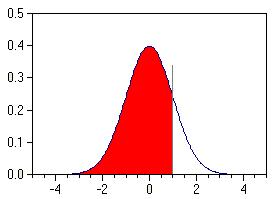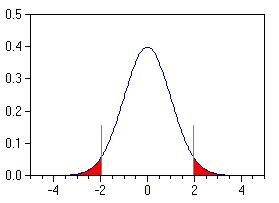# Online Calculator: Standard Normal Distribution by Hasting's best approximation

Use: enter a certain value in the input box, then click "Calculate" to obtain a specified value.

 InputResult z-statstic= F(z)=

where F(z) is the cumulative function of standard normal function, i.e., the area of the red part.

Conversely,one sidedtwo sided Input Result Alpha= critical z-value=

where alpha is level of significance, i.e, the total area of the red part in one-sided or two-sided

The critical z-value is the critical point of standard normal function.

One-sided: Pr(Z>z) = α

Two-sided: Pr(-z<Z) + Pr(Z>z) = α, the outpur shown is the positive critical z-value, as the function is symmetric, another is the negative z-value, i.e., +z and -z

Reference: DistCalc (http://teachme.tuwien.ac.at/simulations/)